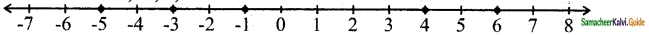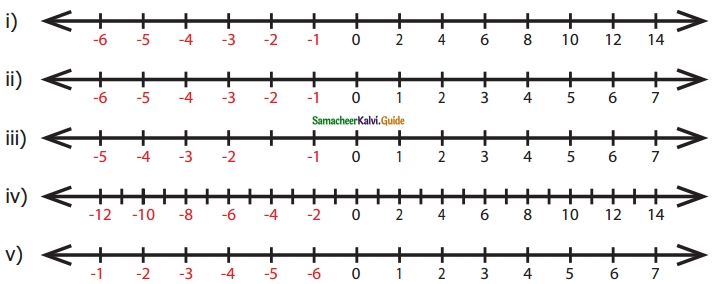Students can download Maths Chapter 2 Integers Ex 2.1 Questions and Answers, Notes, Samacheer Kalvi 6th Maths Guide Pdf helps you to revise the complete Tamilnadu State Board New Syllabus, helps students complete homework assignments and to score high marks in board exams.

## Tamilnadu Samacheer Kalvi 6th Maths Solutions Term 3 Chapter 2 Integers Ex 2.1

Question 1.
Fill in the blanks:
(i) The potable water available at 100 m below the ground level is denoted as ……… m.
(ii) A swimmer dives to a depth of 7 feet from the ground into the swimming pool. The integer that represents this, is ……… feet.
(iii) -46 is to the ……….. of -35 on the number line.
(iv) There are ……… integers from -5 to +5 (both inclusive)
(v) …….. is an integer which is neither positive nor negative.
Solution:
(i) 100
(ii) -7
(iii) left
(iv) 11
(v) 0Question 2.
Say True or False
(i) Each of the integers -18, 6, -12, 0 is greater than -20.
(ii) -1 is to the right of 0.
(iii) -10 and 10 are at equal distance from 1.
(iv) All negative integers are greater than zero.
(v) All whole numbers are integers.
Solution:
(i) True
(ii) False
(iii) False
(iv) False
(v) True

Question 3.
Mark the numbers 4, -3, 6, -1 and -5 on the number line.
Solution:Question 4.
On the number line, which number is
(i) 4 units to the right of -7?
(ii) 5 units to the left of 3?
Solution:
(i) -3
(ii) -2Question 5.
Find the opposite of the following numbers.
(i) 44
(ii) -19
(iii) 0
(iv) -312
(v) 789
Solution:
(i) Opposite of 44 is – 44
(ii) Opposite of-19 is + 19 or 19
(iii) Opposite of 0 is 0
(iv) Opposite of-312 is + 312 or 312
(v) Opposite of 789 is – 789.

Question 6.
If 15 km east of a place is denoted as +15 km, What is the integer that represents 15 km west of it?
Solution:
Opposite of east is west.
∴ If 15 km east is + 15 km, then 15 km west is – 15 km.

Question 7.
From the following number lines, identify the correct and the wrong representations with reason.Solution:
(i) Wrong, Integers are not continuously marked
(ii) Correct, Integers are correctly marked.
(iii) Wrong, Integer -2 is marked wrongly.
(iv) Correct, Integers are marked at equal distance.
(v) Wrong, negative integers marked wrongly.Question 8.
Write all the integers between the given numbers.
(i) 7 and 10
(ii) -5 and 4
(iii) -3 and 3
(iv) -5 and 0
Solution:
(i) 8, 9
(ii) -4, -3, -2, -1, 0, 1, 2, 3
(iii) -2, -1, 0, 1, 2
(iv) -4, -3, -2, -1

Question 9.
Put the appropriate signs as <, > or = in the blank.
(i) -7 ___ 8
(ii) -8 ___ -7
(iii) -999 ___ -1000
(iv) 0 ___ -200
Solution:
(i) <
(ii) <
(iii) >
(iv) =
(v) >Question 10.
Arrange the following integers in ascending order.
(i) -11, 12, -13, 14, -15, 16, -17, 18, -19, -20
(ii) -28, 6, -5, -40, 8, 0, 12, -1, 4, 22
(iii) -100, 10, -1000, 100, 0, -1, 1000, 1, -10
Solution:

(i) -11, 12, -13, 14, -15, 16, -17, 18, -19, -20

• First separating the positive integers 12, 14, 16, 18 and the negative integers -11,-13,-15,-17,-19,-20.
• Then arranging the positive integers in ascending order we get 12, 14, 16, 18 and negative integers in ascending order as -20, -19, -17, -15, -13, -11 4
• Now the ascending order : -20, -19, -17, -15, -13, -11, 12, 14, 16, 18.

(ii) -28, 6, -5, -40, 8, 0, 12, -1, 4, 22

• Positive integers are 6, 8, 12, 4, 22 Negative integers are -28, -5, -40, -1
• Arranging the positive integers in ascending order we get 4, 6, 8, 12, 22 and the negative integers in ascending order -40, -28, -5, -1
• The number 0 is neither positive nor negative and stands in the middle.
• In ascending order : -40, -28, -5, -1, 0, 4, 6, 8,12, 22

(iii) -100, 10, -1000, 100, 0, -1, 1000, 1, -10

• Separating positive integers 10, 100, 1000, 1 and negative integers -100, -1000, -1, -10.
• Now the positive integers in ascending order 1,10,100,1000 and the negative integers in ascending order. -1000, -100, -10, -1
• Also ‘0’ stand in the middle as its is neither positive nor negative.
• ∴ The numbers in ascending order: -1000, -100, -10, -1, 0, 1, 10, 100, 1000.

Question 11.
Arrange the following integers in descending order.
(i) 14, 27, 15, -14, -9, 0, 11, -17
(ii) -99, -120, 65, -46, 78, 400, -600
(iii) 111, -222, 333, -444, 555, -666, 777, -888
Solution:
(i) 27, 15, 14, 11, 0, -9, -14, -17
(ii) 400, 78, 65, -46, -99, -120, -600
(iii) 777, 555, 333, 111, -222, -444, -666, -888Objective Type Questions

Question 12.
There are ……… positive integers from -5 to 6.
(a) 5
(b) 6
(c) 7
(d) 11
Solution:
(c) 7

Question 13.
The opposite of 20 units to the left of 0 is
(a) 20
(b) 0
(c) -20
(d) 40
Solution:
(a) 20Question 14.
One unit to the right of -7 is
(a) +1
(b) -8
(c) -7
(d) -6
Solution:
(d) -6

Question 15.
3 units to the left of 1 is
(a) -4
(b) -3
(c) -2
(d) 3
Solution:
(c) -2Question 16.
The number which determines marking the position of any number to its opposite on a number line is
(a) -1
(b) 0
(c) 1
(d) 10
Solution:
(b) 0